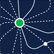# Conference Program

All Times ET

Wednesday, June 8Data Visualization Tools
Wed, Jun 8, 1:15 PM - 2:45 PM
Butler

### Ggdensity: Improved Bivariate Density Visualization in R (310116)

David Kahle, Baylor University Department of Statistical Science
*James Otto, Baylor University Department of Statistical Science

Keywords: Data visualization, density estimation, ggplot2, R

A popular strategy for visually summarizing bivariate data is plotting contours of an estimated density surface. Most commonly, the density is estimated with a kernel density estimator and the plotted contours correspond to equally spaced intervals of the estimated density's height. Notably, this is the case for geom_density_2d() and geom_density_2d_filled() from ggplot2. ggdensity extends ggplot2, providing more interpretable visualizations of density estimates based on highest density regions (HDRs). geom_hdr() and geom_hdr_lines() serve as drop-in replacements for the aforementioned ggplot2 functions, plotting density contours that are chosen to be inferentially relevant. By default, they plot the smallest regions containing 50%, 80%, 95%, and 99% of the estimated density (the HDRs). This results in very interpretable graphics, conveying more information than arbitrary density contours. ggdensity allows for the plotting of contours of densities estimated via methods other than the standard kernel density estimator. Densities can also be estimated by histograms, frequency polygons, and fitting a parametric bivariate normal model. Also included are the functions geom_hdr_fun() and geom_hdr_fun_lines() for plotting HDRs of user-specified probability density functions. This allows for the plotting of a much larger class of HDR estimators than the four available for geom_hdr(). Users can specify and estimate arbitrary parametric models, providing the resulting pdf estimates to geom_hdr_fun() for contouring.